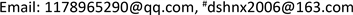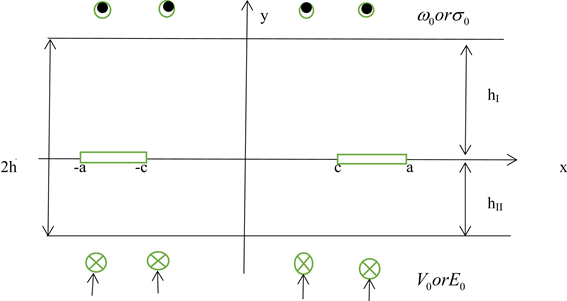﻿ 傅里叶变换在材料中的应用 The Application of Fourier Transform in Materials

Pure Mathematics
Vol.08 No.03(2018), Article ID:25135,11 pages
10.12677/PM.2018.83037

The Application of Fourier Transform in Materials

Xiang Mu*, Shenghu Ding#

School of Mathematical Statistics, Ningxia University, Yinchuan NingxiaReceived: May 6th, 2018; accepted: May 20th, 2018; published: May 29th, 2018ABSTRACT

From the perspective of modern mathematics, the Fourier transform is a special integral transform. It can express a certain function that satisfies certain conditions as a linear combination or integration of sine basis functions. In different research fields, Fourier transforms have a variety of different variations, such as a continuous Fourier transform and a discrete Fourier transform. This article mainly introduces the application of Fourier transform in dealing with the performance, contact and fracture of piezoelectric materials and thermoelectric materials.

Keywords:Fourier Transform, Piezoelectric Materials, Thermoelectric Materials, Singular Integral Equation, Dual Integral Equations1. 引言

Kelvin说，傅里叶理论不仅是现代分析中最美妙的结果之一，而且可以说，它为现代物理中每一个深奥问题的处理提供了一件必不可少的工具。随着近代物理的飞速发展，越来越多的实际问题需要用数学理论来解决。傅里叶变换是一类重要的积分变换，而积分变换能够将分析运算转换为代数运算，正是由于积分变换这一特性，在微分方程、偏微分方程、积分方程的求解中成为重要的方法之一。本文主要综述了傅里叶变换在压电材料、热电材料中的应用。

2. 傅里叶变换

2.1. 傅里叶变换的提出

2.2. 傅里叶变换的数学意义

$f\left(t\right)$ 是t的周期函数，如果t满足狄利克雷条件：在一个以2T为周期内 $f\left(X\right)$ 连续或只有有限个第一类间断点，附 $f\left(X\right)$ 单调或可划分成有限个单调区间，则 $f\left(X\right)$ 以2T为周期的傅里叶级数收敛，和函数 $S\left(X\right)$ 也是以2T为周期的周期函数，且在这些间断点上，函数是有限值；在一个周期内具有有限个极值点；绝对可积  。则有(1)式成立

$F\left(\omega \right)=F\left[f\left(t\right)\right]=\underset{-\infty }{\overset{\infty }{\int }}f\left(t\right){\text{e}}^{-jwt}\text{d}t$ (1)

$f\left(t\right)={F}^{-1}\left[F\left(\omega \right)\right]=\frac{1}{\text{2π}}\underset{-\infty }{\overset{\infty }{\int }}F\left(\omega \right){\text{e}}^{jwt}\text{d}\omega$ (2)

3. 傅里叶变换在材料中的应用

3.1. 傅里叶变换在压电材料中的应用

3.1.1. 由傅里叶变换得到奇异积分方程

H.S. Nan，B.L. Wang  通过考虑残余表面应力对裂纹表面的影响，研究压电纳米材料的纳米级裂纹问题时，根据解决压电介质裂纹问题的方法，利用傅里叶积分变换和特征方程导出位移和势函数，通过引入满足边界条件的辅助函数，最终得到了奇异积分方程组，得到了裂纹尖端场。Jie Su，Liao-Liang Ke  等首次尝试对刚性圆柱形冲头下压电半平面微动接触进行理论研究时，利用Fourier积分变换技术和叠加定理，将问题简化为一组耦合的Cauchy奇异积分方程。Zengtao Chen  在研究尺寸对冲击载荷作用下压电带裂纹扭结的影响时，应用傅里叶变换和拉普拉斯变换将混合模式裂纹问题(模式I和II)减少为奇异积分方程组。Yue-Ting Zhou  在研究三角形和圆柱形冲头对单斜压电材料摩擦滑动接触问题时，通过傅里叶变换将混合边值问题简化为第二类Cauchy型奇异积分方程。基于简化奇异积分方程的精确解，得到了各种表面应力和电位移的闭合表达式。钟献词  在积分变换方法求解压电带形中静止或运动的共线裂纹问题时，在电渗透裂纹和非电渗透型的裂纹假设下，利用Fourier积分变换把相应的混合边值问题转化为求解奇异积分方程。陈建  研究压电功能梯度材料层中平行于边界的动态反平面裂纹问题时，采用拉普拉斯、傅里叶积分变换和位错函数法将问题简化为Cauchy奇异积分方程，最后给出数值结果。贾洪波  在研究正交各向异性压电材料中III型裂纹对SH波的散射问题时，使用Fourier变换并引入位错密度函数，将混合边值问题转化为奇异积分方程。魏苓彦  在研究正交各向异性压电材料中III型裂纹受冲击力电载荷作用时的瞬态断裂问题，基于电可渗透裂纹模型，采用积分变换技术和数值求解奇异积分方程，最后反演得到了动态应力强度因子的表达式。B. Gu  等在研究机械和电气作用下不同压电层间绝缘裂纹的瞬态响应问题时，利用拉普拉斯、傅里叶积分变换和Cauchy奇异积分方程方法，在电绝缘的边界条件下，解决了两个有限宽度的不同压电层受到机电冲击的界面裂纹面内问题。刘俊俏  用奇异积分方程方法研究SH波和压电压磁材料中周期裂纹的相互作用。根据电磁弹性材料的控制微分方程，通过傅立叶积分变换，将问题转化为Hilbert核的奇异积分方程组。李永东  在研究压电界面电极的应力强度因子时，建立了压电界面电极的力学模型，利用有限傅里叶变换方法将相应的力电耦合问题描述为奇异积分方程，并得到了界面应力及其强度因子的解析解。常志艳  在研究压电材料全平面含平行线裂纹问题时，通过引入势函数将原来获得的压电方程整理为较简单的偏微分方程组，再利用算子作用和Fourier变换等数学工具将偏微分方程转化为常微分方程求解。赵明皞  等运用广义不连续位移边界元法研究二维压电半导体材料在多场耦合环境下的断裂问题，利用傅里叶变换，给出二维压电半导体的广义不连续位移基本解。邢时超  研究了含半绝缘裂纹的压电条上、下表面在受到不同温度与电载荷作用时的断裂问题。基于压电材料的本构方程、热控制方程和边界条件，并结合Fourier变换和奇异积分公式法，获得了压电板条内温度场和应力场的解。杨昌锦，李尧臣  在进行有限厚压电层表面金属电极脱层的屈曲研究时，用Fourier积分变换，界面连续条件和上、下电极表面的边界条件将问题归为第二类Cauchy型奇异积分方程组。乐金朝  等使用Fourier积分变换，对非对称载荷作用下的Griffith裂纹问题进行了研究，利用裂纹两侧的位移和应力联结条件，将问题归结为一组带Cauchy核的奇异积分方程，通过Cauchy反演求得了问题的精确解。彭达仁  等在研究层状介质垂直界面有限裂纹问题时，运用傅里叶变换及引用

${c}_{44}{\nabla }^{2}\omega +{e}_{15}{\nabla }^{2}\varphi =0,\text{\hspace{0.17em}}\text{\hspace{0.17em}}{e}_{15}{\nabla }^{2}\omega -{\epsilon }_{11}{\nabla }^{2}\varphi =0$ (3)

${\nabla }^{2}\omega =0,\text{}{\nabla }^{2}\varphi =0$ (4)

${\sigma }_{xz}={c}_{44}{\gamma }_{xz}-{e}_{15}{E}_{x},\text{}{\sigma }_{yz}={c}_{44}{\gamma }_{yz}-{e}_{15}{E}_{y}$ (5)

${D}_{x}={e}_{15}{\gamma }_{xz}+{\epsilon }_{11}{E}_{x},\text{}{D}_{y}={e}_{15}{\gamma }_{yz}+{\epsilon }_{11}{E}_{y}$ (6)

${\gamma }_{xz}=\frac{\partial \omega }{\partial x}\text{},\text{}{\gamma }_{yz}=\frac{\partial \omega }{\partial y}$ (7)

${E}_{x}=\frac{\partial \phi }{\partial x},\text{}{D}_{y}={e}_{15}{\gamma }_{yz}+{\epsilon }_{11}{E}_{y}$ (8)Figure 1. Piezoelectric material with two collinear cracks

 (9)

${D}_{y}^{I}\left(x,{0}^{+}\right)={D}_{y}^{II}\left(x,{0}^{-}\right)\text{}c<|x| (10)

${\sigma }_{yz}^{I}\left(x,{0}^{+}\right)={\sigma }_{yz}^{II}\left(x,{0}^{-}\right)=0\text{}c<|x| (11)

${\phi }^{I}\left(x,{0}^{+}\right)={\phi }^{II}\left(x,{0}^{-}\right)\text{}|x|a$ (12)

${D}_{y}^{I}\left(x,{0}^{+}\right)={D}_{y}^{II}\left(x,{0}^{-}\right)\text{}|x|a$ (13)

${\sigma }_{yz}^{I}\left(x,{0}^{+}\right)={\sigma }_{yz}^{II}\left(x,{0}^{-}\right)\text{}|x|a$ (14)

${\omega }^{I}\left(x,{0}^{+}\right)={\omega }^{II}\left(x,{0}^{-}\right)\text{}|x|a$ (15)

$\begin{array}{l}{\sigma }_{yz}^{I}\left(x,{h}_{I}\right)={\sigma }_{yz}^{II}\left(x,-{h}_{II}\right)={\sigma }_{0},\text{}-\infty (16)

${\int }_{0}^{\infty }\left({\nabla }^{2}\omega \right)\mathrm{cos}\left(\xi x\right)\text{d}x=\left(\frac{{\text{d}}^{\text{2}}}{\text{d}{y}^{2}}-{\xi }^{2}\right)\overline{\omega }\left(\xi ,y\right)$ (17)

$\omega \left(x,y\right)=\frac{2}{\text{π}}{\int }_{0}^{\infty }\overline{\omega }\left(\xi ,y\right)\mathrm{cos}\left(\xi x\right)\text{d}\xi$ (18)

$\overline{\omega }\left(\xi ,y\right)=A\left(\xi \right)\mathrm{exp}\left(-\xi y\right)+B\left(\xi \right)\mathrm{exp}\left(\xi y\right)$ (19)

$\omega \left(x,y\right)=\frac{2}{\text{π}}{\int }_{0}^{\infty }\left[A\left(\xi \right)\mathrm{exp}\left(-\xi y\right)+B\left(\xi \right)\mathrm{exp}\left(\xi y\right)\right]\mathrm{cos}\left(\xi x\right)\text{d}\xi +{A}_{0}y$ (20)

$\phi \left(x,y\right)=\frac{2}{\text{π}}{\int }_{0}^{\infty }\left[C\left(\xi \right)\mathrm{exp}\left(-\xi y\right)+D\left(\xi \right)\mathrm{exp}\left(\xi y\right)\right]\mathrm{cos}\left(\xi x\right)\text{d}\xi +{C}_{0}y$ (21)

$\begin{array}{c}{\sigma }_{yz}\left(x,y\right)=\frac{2{c}_{44}}{\text{π}}{\int }_{0}^{\infty }\left(-\xi \right)\left[A\left(\xi \right)\mathrm{exp}\left(-\xi y\right)-B\left(\xi \right)\mathrm{exp}\left(\xi y\right)\right]\mathrm{cos}\left(\xi x\right)\text{d}\xi +{A}_{0}{c}_{44}+{C}_{0}{e}_{15}\\ \text{\hspace{0.17em}}\text{\hspace{0.17em}}+\frac{2{e}_{15}}{\text{π}}{\int }_{0}^{\infty }\left(-\xi \right)\left[C\left(\xi \right)\mathrm{exp}\left(-\xi y\right)-D\left(\xi \right)\mathrm{exp}\left(\xi y\right)\right]\mathrm{cos}\left(\xi x\right)\text{d}\xi ,\end{array}$ (22)

$\begin{array}{c}{D}_{y}\left(x,y\right)=\frac{2{e}_{15}}{\text{π}}{\int }_{0}^{\infty }\left(-\xi \right)\left[A\left(\xi \right)\mathrm{exp}\left(-\xi y\right)-B\left(\xi \right)\mathrm{exp}\left(\xi y\right)\right]\mathrm{cos}\left(\xi x\right)\text{d}\xi +{A}_{0}{e}_{15}-{C}_{0}{\epsilon }_{11}\\ \text{\hspace{0.17em}}\text{\hspace{0.17em}}-\frac{2{\epsilon }_{11}}{\text{π}}{\int }_{0}^{\infty }\left(-\xi \right)\left[C\left(\xi \right)\mathrm{exp}\left(-\xi y\right)-D\left(\xi \right)\mathrm{exp}\left(\xi y\right)\right]\mathrm{cos}\left(\xi x\right)\text{d}\xi ,\end{array}$ (23)

$\frac{4}{\text{π}}{\int }_{c}^{a}\Delta {{\omega }^{\prime }}_{1}\left(t\right)\text{d}t{\int }_{0}^{\infty }\frac{\mathrm{tanh}\left({h}_{I}\xi \right)\mathrm{tanh}\left({h}_{II}\xi \right)}{\mathrm{tanh}\left({h}_{I}\xi \right)+\mathrm{tanh}\left({h}_{II}\xi \right)}\mathrm{cos}\left(x\xi \right)\mathrm{sin}\left(t\xi \right)\text{d}\xi =-\frac{{\sigma }_{0}}{{c}_{44}}\text{}c (24)

$\frac{2}{\text{π}}{\int }_{0}^{\infty }\mathrm{cos}\left(x\xi \right)\mathrm{sin}\left(t\xi \right)\text{d}\xi =\frac{1}{\text{π}}\left(\frac{\text{1}}{\text{t}+x}+\frac{1}{t-x}\right)$

$\frac{2}{\text{π}}{\int }_{c}^{a}\frac{\Delta {{\omega }^{\prime }}_{1}\left(t\right)}{t-x}\text{d}t+\frac{2}{\text{π}}{\int }_{c}^{a}\Delta {{\omega }^{\prime }}_{1}\left(t\right){k}^{\left(1\right)}\left(t,x,{h}_{I},{h}_{II}\right)\text{d}t=-\frac{{\tau }^{\left(1\right)}}{{c}_{44}}$ (25)

${k}^{\left(1\right)}\left(t,x,{h}_{I},{h}_{II}\right)={\int }_{0}^{\infty }2\left[\frac{\mathrm{tanh}\left({h}_{I}\xi \right)\mathrm{tanh}\left({h}_{II}\xi \right)}{\mathrm{tanh}\left({h}_{I}\xi \right)+\mathrm{tanh}\left({h}_{II}\xi \right)}-1\right]\mathrm{cos}\left(x\xi \right)\mathrm{sin}\left(t\xi \right)\text{d}\xi +\frac{1}{t+x}$

3.1.2. 由傅里叶变换得到对偶积分方程组

Keqiang Hu  等研究Dugdale型裂纹沿着两种不同的压电材料的界面移动的问题时，应用傅里叶变换将裂纹的混合边值问题转化为对偶积分方程，得到了精确求解。Yi-Ze Wang  在讨论残余极化和周围介质中麦克斯韦应力对两种不同压电材料之间的反平面界面裂纹的影响时，通过傅里叶变换和它的逆变换，它被转化成两对双积分方程。推导了应力强度因子和电位移的闭合解。Jeong Woo Shin  采用积分变换方法，分析了两种不同功能梯度压电材料界面裂纹在反平面剪切冲击载荷作用下的瞬态响应，用拉普拉斯变换和傅里叶变换将问题简化为两组对偶积分方程，然后将其表示为第二类的Fredholm积分方程。R. Bagheri  等在研究功能梯度压电条中多个运动裂纹的解析解时，利用复数傅里叶变换获得了变换的位移和应力场。马旭  通过对裂纹的无限长功能梯度/压电材料层合的SH波散射问题的研究，用Fourier积分变换把混合边值问题的求解转化为对偶积分方程，然后利用Copson方法将得到的对偶积分方程转化为Fredholm积分方程再进行数值求解。郭玉彬  在忽略界面上裂纹尖端裂纹面相互叠入的前提下，讨论了功能梯度材料与压电材料拼接界面上的反平面运动裂纹问题。通过Fourier积分变换，将混合边值问题转化为对偶积分方程，并利用Copson-Sih方法将对偶积分方程转化为第二类Fredholm积分方程进行求解，给出了反平面位移、电势及应力分量的解析表达式。杜勇峰、王建国  采用Schmidt方法研究条形压电材料和弹性材料的界面III型裂纹问题，主要探讨条形压电材料和弹性材料受反平面剪应力和平面电位移作用的情形；通过Fourier积分变换和界面裂纹位移差的车贝雪夫多项式假设，并利用Schmidt方法得到数值解。边文凤  在研究压电材料I型裂纹动态问题的对偶方程组及其求解的情况时，采用Laplace变换、半无限对称Fourier 正弦变换和Fourier余弦变换，对用势函数表示的压电材料基本微分方程进行变换和初步求解；然后通过Fourier反演和引入边界条件，建立了二维压电材料动态裂纹问题的对偶方程组，曾云  用含有电场梯度效应的电弹性体理论分析了压电体中的反平面裂纹问题。利用Fourier积分变换方法，将相应的复合边值问题转化为对偶积分方程组。求解这些方程组，获得了裂纹尖端的强度因子和能量释放率。刘海涛  在研究压电材料中矩形裂纹材料的三维断裂问题时，通过二维Fourier变换技术将此类问题的求解转化为三对以矩形裂纹上下面的位移间断函数为未知变量的对偶积分方程，并采用Schmidt方法进行数值求解。胡克强  在研究矩形压电体中反平面裂纹的电弹性场的问题时，利用Fourier积分变换和Fourier正弦级数将电绝缘型裂纹问题化为对偶积分方程，并进一步归结为易于求解的第二类Fredholm积分方程。哈元军  在讨论无限长功能梯度压电板中双裂纹尖端热响应分析时，用Fourier积分变换把混合边值问题转化为以裂纹面上位移为未知量的对偶积分方程，利用Schmidt方法对其求解。杨娟  利用积分变换及奇异积分方程技术研究电磁复合材料底层处裂纹对SH波散射问题。假定裂纹面的边界条件为电渗透性，通过Fourier余弦变化将问题转化为对偶积分方程，并利用Copson方法将对偶积分方程转化为第二类Fredholm积分方程。冯文杰  等在研究加层压电条界面裂纹的稳态扩展时，应用Fourier积分变换将问题化为以第二类Fredholm积分方程表示的对偶积分方程，导出了相应的动应力强度因子表达式。陆万顺  等分析了功能梯度压电材料中裂纹尖端的热应力，针对考虑的问题，通过Fourier积分变换把混合边值问题的求解转化为裂纹面上位移间断为未知量的对偶积分方程，然后利用Schmidt方法来求解。胡克强  等基于三维弹性理论和压电理论，研究了功能梯度压电板条中的电渗透型运动裂纹问题。利用Fourier积分变换方法，将混合边值问题化为对偶积分方程，并进一步归结为易于数值求解的第二类Fredholm积分方程。曾云  等用含有电场梯度效应的电弹性体理论分析了压电体中的III型裂纹问题，利用傅里叶积分变换方法，将相应的混合边值问题转化为对偶积分方程组，求解这些方程组，得到了裂纹尖端处的电场强度因子。

3.2. 傅里叶积分变换在热电材料中的应用

Magdy A. Ezzat  在修改傅里叶定律的热电MHD时，构建了一个具有分数阶导数和热电性质的热方程的数学模型。在边界层框架内的控制耦合方程应用于斯托克斯在存在垂直于板的横向磁场的情况下热源分布的无限板上的导电流的第一个问题，拉普拉斯变换和状态空间方法技术被用来获得解决方案。拉普拉斯变换是利用变换的复数反演公式和傅里叶展开技术得到的。Magdy A. Ezzat和Hamd M. Youssef  在研究斯托克斯对于具有热电性质的导电微极流体第一个问题时，将边界层模型框架内的控制耦合方程应用于斯托克斯热源的第一个问题。拉普拉斯变换和傅立叶变换技术被用来获得解决方案。傅里叶变换的倒数是分析获得的。拉普拉斯变换是利用变换的复数反演公式和傅里叶展开技术得到的。该方法也在Magdy A. Ezzat  在研究具有分数导数热传递的热电MHD非牛顿流体中得到应用。B.L.Wang    等在研究裂纹对热电转换效率的影响时，利用傅里叶变换技术和奇异积分方程方法，得到了电势和温度场分布的解析表达式，得到了裂纹尖端的电流密度和强度因子，有助于确定热电转换效率。以B.L.Wang文章为例，在研究裂纹对热电转换效率的影响时，为了使计算过程简洁容易，引进一总的势函数F，F表示总的电势，通过对原有的本构方程进行代入和化简，可得到T和温度场T的表达式

${\nabla }^{2}F=0$ (26)

$k{\nabla }^{2}T+\sigma {\left(\nabla F\right)}^{2}=0$ (27)

$F\left(x,y\right)\frac{{F}_{10}-{F}_{20}}{2h}y+\frac{{F}_{10}+{F}_{20}}{2}=+\sum _{n=1}^{\infty }\sum _{m=1}^{2}\mathrm{sin}\left({s}_{n}y\right)\mathrm{exp}\left({s}_{n}x{\lambda }_{m}\right){A}_{mn}$ $+\frac{2}{\text{π}}{\int }_{0}^{\infty }\sum _{m=1}^{2}\mathrm{cos}\left(sx\right)\mathrm{exp}\left(sy{\gamma }_{m}\right){B}_{m}ds$ (28)

${j}_{ey}\left(x,y\right)$ $={j}_{e0}-\sigma \sum _{n=1}^{\infty }\sum _{m=1}^{2}{s}_{n}\mathrm{cos}\left({s}_{n}y\right)\mathrm{exp}\left({s}_{n}x{\lambda }_{m}\right){A}_{mn}-\frac{2\sigma }{\text{π}}\sum _{m=1}^{2}{\gamma }_{m}{\int }_{0}^{\infty }s\mathrm{cos}\left(sx\right)\mathrm{exp}\left(sy{\gamma }_{m}\right){B}_{m}\text{d}s$ (29)

${j}_{ex}\left(x,y\right)=$ $-\sigma \sum _{n=1}^{\infty }\sum _{m=1}^{2}{s}_{n}{\lambda }_{m}\mathrm{sin}\left({s}_{n}y\right)\mathrm{exp}\left({s}_{n}x{\lambda }_{m}\right){A}_{mn}+\frac{2\sigma }{\text{π}}\sum _{m=1}^{2}{\int }_{0}^{\infty }s\mathrm{sin}\left(sx\right)\mathrm{exp}\left(sy{\gamma }_{m}\right){B}_{m}\text{d}s$ (30)

${f}_{0}\left(x\right)=\left\{\begin{array}{l}\frac{\partial }{\partial x}\left[F\left(x,+0\right)-F\left(x,-0\right)\right],\text{\hspace{0.17em}}|x|\le a\\ 0,\text{}\text{\hspace{0.17em}}\text{\hspace{0.17em}}|x|>a\end{array}$ (31)

${B}_{m}={N}_{m}\left(s\right){\int }_{0}^{a}{f}_{0}\left(r\right)\mathrm{sin}\left(sr\right)\text{d}r$ (32)

${N}_{1}\left(s\right)=-\frac{1}{s\left[1-\mathrm{exp}\left(2sh{\gamma }_{1}\right)\right]},\text{\hspace{0.17em}}{N}_{2}\left(s\right)=-\mathrm{exp}\left(2sh{\gamma }_{1}\right){N}_{1}\left(s\right)$

${A}_{1n}={A}_{2n}=-\frac{1}{2h}{\int }_{0}^{a}{f}_{0}\left(r\right)v\left({s}_{n},r\right)\text{d}r$ (33)

$\frac{1}{\text{π}}{\int }_{-a}^{a}{f}_{0}\left(r\right)\left[\frac{1}{r-x}+{M}_{0}\left(r,x\right)\right]\text{d}r=\frac{{j}_{e0}}{\sigma }$ (34)

${\int }_{-a}^{a}{f}_{0}\left(r\right)\text{d}r=0$ (35)

4. 结束语

The Application of Fourier Transform in Materials[J]. 理论数学, 2018, 08(03): 278-288. https://doi.org/10.12677/PM.2018.83037

1. 1. 吕诚. 积分变换教学中的深入浅出式方法研究[J]. 鸡西大学学报, 2015(10): 27-29.

2. 2. Nan, H.S. and Wang, B.L. (2013) Effect of Crack Face Residual Surface Stress on Nanoscale Fracture of Piezoelectric Materials. Engineering Fracture Mechanics, 110, 68-80. https://doi.org/10.1016/j.engfracmech.2013.08.002

3. 3. Su, J., Ke, L.-L. and Wang, Y.-S. (2015) Two-Dimensional Fretting Contact Analysis of Piezoelectric Materials. International Journal of Solids and Structures, 73-74, 41-45. https://doi.org/10.1016/j.ijsolstr.2015.07.026

4. 4. Hu, K.Q. and Chen, Z.T. (2013) Size Effect on Crack Kinking in a Piezoe-lectric Strip under Impact Loading. Mechanics of Materials, 61, 60-72. https://doi.org/10.1016/j.mechmat.2013.03.001

5. 5. Zhou, Y.-T. and Lee, K.Y. (2014) Investigation of Frictional Sliding Contact Problems of Triangular and Cylindrical Punches on Monoclinic Piezoelectric Materials. Mechanics of Materials, 69, 237-250. https://doi.org/10.1016/j.mechmat.2013.10.008

6. 6. 钟献词. 积分变换方法求解压电带形中静止或运动的共线裂纹问题[D]: [硕士学位论文]. 长沙: 湖南师范大学, 2004.

7. 7. 陈建, 刘正兴. 压电功能梯度材料层反平面裂纹瞬态问题的研究[J]. 上海交通大学学报, 2003, 37(4): 527-531.

8. 8. Wang, B.L. and Han, J.C. (2010) Thermal Conduction in Bi-Layer Materials with an Interfacial Inclusion. Philosophical Magazine Letters, 90, 241-249. https://doi.org/10.1080/09500831003630740

9. 9. 贾洪波. 正交各向异性压电、弹性和压磁复合介质中III型裂纹对SH波的散射[D]: [硕士学位论文]. 石家庄: 石家庄铁道大学,2015.

10. 10. 魏苓彦. 正交各向异性压电材料中III型裂纹的瞬态响应[D]: [硕士学位论文]. 石家庄: 石家庄铁道大学,2015.

11. 11. Gu, B., Yu, S.W. and Feng, X.Q. (2002) Transient Response of an Insulating Crack between Dissimilar Piezoelectric Layers under Mechanical and Electrical Impacts. Archive of Applied Mechanic, 72, 615-629. https://doi.org/10.1007/s00419-002-0239-4

12. 12. 刘俊俏, 李星. 压电压磁材料中周期裂纹对SH波的散射[J]. 工程数学学报, 2011, 28(4): 470-474.

13. 13. 李永东, 张丙喜, 张男. 压电界面电极的应力强度因子[J]. 装甲兵工程学院学报, 2009, 23(2): 88-91.

14. 14. 常志艳. 压电材料全平面含平行线裂纹问题研究[D]: [硕士学位论文]. 大连: 大连海事大学, 2017.

15. 15. 赵明皞, 潘一博, 徐广涛, 范翠英. 二维压电半导体的断裂问题分析[C]//中国机械工程学会. 全国疲劳与断裂学术会议, 2016.

16. 16. 邢时超. 热–力–电载荷作用下压电材料的断裂研究[D]: [硕士学位论文]. 南京: 南京航空航天大学, 2013.

17. 17. 杨昌锦, 李尧臣. 有限厚压电层表面金属电极脱层的屈曲研究[J]. 固体力学学报, 2009, 30(1): 28-34.

18. 18. 乐金朝, 王复明, 刘文廷. 非对称载荷作用的Griffith裂纹问题的Fourier积分变换解法[J]. 郑州工业大学学报(工学版), 1996, 17(3): 20-25.

19. 19. 彭达仁, 张起森, 杨光松. 层状介质垂直界面有限裂纹问题的积分变换解[J]. 固体力学学报, 1998, 19(2): 148-155.

20. 20. Ueda, S. (2006) The Crack Problem in Piezoelectric Strip under Thermoelectric Loading. Journal of Thermal Stress, 29, 295-316. https://doi.org/10.1080/01495730500360450

21. 21. Ueda, S. (2006) Transient Response of a Cracked Piezoelectric Strip under Thermoelectric Loading. Journal of Thermal Stress, 29, 973-994. https://doi.org/10.1080/01495730600710208

22. 22. Tani, Y. (2008) Thermal Stress Intensity Factors for Two Coplanar Cracks in a Piezoelectric Strip. Journal of Thermal Stress, 31, 403-415. https://doi.org/10.1080/01495730701876809

23. 23. Ueda, S. (2007) Effects of Crack Surface Conductance on Intensity Factors for a Functionally Graded Piezoelectric Material under Thermal Load. Journal of Thermal Stress, 30, 731-752. https://doi.org/10.1080/01495730701212831

24. 24. Ikawa, K. (2008) Thermoelectromechanical Interaction between Two Parallel Cracks in a Piezoelectric Strip. Journal of Thermal Stress, 31, 311-330. https://doi.org/10.1080/01495730701738314

25. 25. Ueda, S. and Hatano, H. (2012) T-Shaped Crack in a Piezoelectric Material under Thermo-Electro-Mechanical Loadings. Journal of Thermal Stress, 35, 12-29. https://doi.org/10.1080/01495739.2011.616799

26. 26. Ueda, S. and Kondo, H. (2008) Transient Intensity Factors for a Parallel Crack in a Plate of a Functionally Graded Piezoelectric Material under Thermal Shock Loading Conditions. Journal of Thermal Stress, 31, 211-232. https://doi.org/10.1080/01495730701215313

27. 27. Ueda, S. (2004) Thermally Induced Fracture of a Functionally Graded Pie-zoelectric Layer. Journal of Thermal Stress, 27, 291-309. https://doi.org/10.1080/01495730490427555

28. 28. Ueda, S. and Ashida, Y. (2009) Infinite Row of Parallel Cracks in a Functionally Graded Piezoelectric Material Strip under Mechanical and Transient Thermal Loading. Journal of Thermal Stress, 32, 1103-1125. https://doi.org/10.1080/01495730903248955

29. 29. Ueda, S. and Ishii, A. (2008) Thermoelectromechanical Response of a Pie-zoelectric Strip with Two Parallel Cracks of Different Lengths. Journal of Thermal Stress, 31, 976-990. https://doi.org/10.1080/01495730802250557

30. 30. Hu, K., Fu, J. and Yang, Z. (2014) Moving Dugdale Type Crack along the Interface of Two Dissimilar Piezoelectric Materials. Theoretical and Applied Fracture Mechanics, 74, 157-163. https://doi.org/10.1016/j.tafmec.2014.09.004

31. 31. Wang, Y.-Z. (2015) Influences of the Remanent Polarization and Maxwell Stress in Surrounding Medium on a Moving Anti-Plane Crack between Two Dissimilar Piezoelectric Solids. Theoretical and Applied Fracture Mechanics, 80, 253-258. https://doi.org/10.1016/j.tafmec.2015.06.004

32. 32. Shin, J.W., Lee, Y.-S. and Kim, S.J. (2013) An Interface Crack in a Functionally Graded Piezoelectric Bi-Layer under Anti-Plane Shear Impact. Acta Mechanica, 224, 867-879. https://doi.org/10.1007/s00707-012-0794-x

33. 33. Bagheri, R., Ayatollahi, M. and Mousavi, S.M. (2015) Analysis of Cracked Piezoelectric Layer with Imperfect Non-Homogeneous Orthotropic Coating. International Journal of Mechanical Science, 93, 93-101. https://doi.org/10.1016/j.ijmecsci.2014.11.025

34. 34. 马旭. 功能梯度/压电材料中裂纹对SH波的散射问题[J]. 苏州科技学院学报(自然科学版), 2009, 26(2): 44-50.

35. 35. 郭玉彬. 功能梯度材料与压电材料拼接界面上的反平面运动裂纹[J]. 宁夏大学学报, 2008, 29(3): 193-197.

36. 36. 杜勇峰, 王建国. 条形压电材料和弹性材料Ⅲ型界面裂纹分析[J]. 合肥工业大学学报, 2015, 28(12): 1574-1577.

37. 37. 边文凤. 压电材料I型裂纹动态问题的对偶方程组及其求解[J]. 应用数学和力学, 2007, 28(6): 651-658.

38. 38. 曾云, 胡元太, Yang Jiashi. 压电反平面裂纹问题中的电场梯度效应[J]. 土木工程与管理学报, 2005, 22(s1): 31-35.

39. 39. 刘海涛. 几类含矩形裂纹材料的三维断裂问题研究[D]: [博士学位论文]. 哈尔滨: 哈尔滨工业大学, 2015.

40. 40. 胡克强. 矩形压电体中反平面裂纹的电弹性场[J]. 力学季刊, 2006, 27(1): 45-51.

41. 41. 哈元军. 具周期裂纹半无限大功能梯度压电材料的热应力分析[J]. 宁夏师范学院学报, 2013, 34(6): 10-15.

42. 42. 杨娟. 压电拼接电磁复合材料中裂纹对SH波的散射[J]. 振动与冲击, 2014, 33(20): 192-197.

43. 43. 冯文杰, 张会斌, 王丽群. 加层压电条界面裂纹的稳态扩展[J]. 工程力学, 2004, 21(4): 112-117.

44. 44. 陆万顺, 马旭, 李星. 功能梯度压电材料中裂纹尖端热应力分析[J]. 宁夏大学学报, 2012, 33(2): 125-129.

45. 45. 胡克强, 仲政, 李国强. 功能梯度压电板条中的电渗透型运动裂纹[J]. 同济大学学报, 2004, 32(12): 1631-1636.

46. 46. 曾云, 胡元太, Yang Jia-shi. III型压电裂纹尖端的电场梯度效应分析[J]. 宁波大学学报, 2004, 17(sup.): 1-5.

47. 47. 刘静, 李敬锋. 热电材料的应用及研究进展[J]. 新材料产业, 2004(8): 49-53.

48. 48. Ezzat, M.A. (2011) Thermoelectric MHD with Modified Fourier’s Law. International Journal of Thermal Sciences, 50, 449-455. https://doi.org/10.1016/j.ijthermalsci.2010.11.005

49. 49. Ezzat, M.A. and Youssef, H.M. (2010) Stokes’ First Problem for an Electro-Conducting Micropolar Fluid with Thermoelectric Properties. Canadian Journal of Physics, 88, 35-48. https://doi.org/10.1139/P09-100

50. 50. Ezzat, M.A. (2010) Thermoelectric MHD Non-Newtonian Fluid with Fractional Derivative Heat Transfer. Physica B: Condensed Matter, 405, 4188-4194. https://doi.org/10.1016/j.physb.2010.07.009

51. 51. Zhang, A.B., Wang, B.L., Wang, J., Du, J.K., Xie, C. and Jin, Y.A. (2017) Thermodynamics Analysis of Thermoelectric Materials: Influence of Cracking on Efficiency of Thermoelectric Conversion. Applied Thermal Engineering, 127, 1442-1450. https://doi.org/10.1016/j.applthermaleng.2017.08.154

52. 52. Zhang, A.B., Wang, B.L., Wang, J., Du, J.K. and Xie, C. (2017) Effect of Cracking on the Thermoelectric Conversion Efficiency of Thermoelectric Materials. Journal of Applied Physics, 121, Article ID: 04510. https://doi.org/10.1063/1.4974848